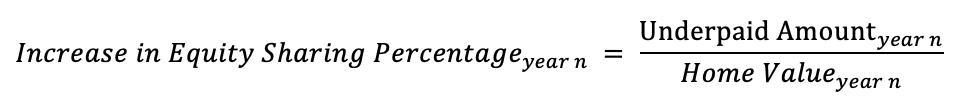Vessel Tutorial
Share
Explore
Tutorial of Math

#Conversion to Equity

At the property owner's discretion, the annual payment may be "paid" in part or in full with additional equity rights granted to the investors. The amount "underpaid" (i.e. the amount owed minus the amount paid with cash) is converted to additional equity sharing rights at the end-of period interim home value.
Equity Sharing Increase at year n = Underpaid Amount at year n / Interim Home Value at Year n
Next Topic:
Adjust the sliders below to try it out.
Input:
Income Payment owed
\$
00000
2500
\$
0000
1100
Interim Property Value at year end
\$
0000000
550000
Output:
Underpaid Amount
\$
2500
- \$
1100
= \$
1400
Equity Sharing Percentage Increase
= \$
1400
/ \$
550000
=
0.25
%
So, if at the beginning of one period, the Equity Sharing Percentage were
10
%, then the equity sharing percentage would be
10
% +
0.25
% =
10.25
% at the beginning of the next period, which would be used to calculate next period's annual fee and also used to calu
Next Topic: